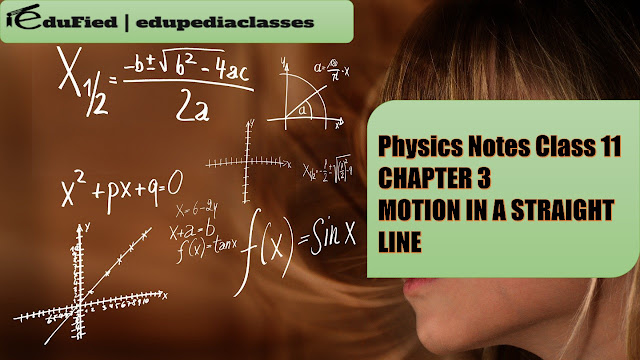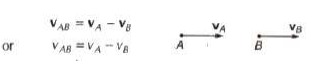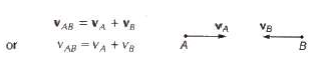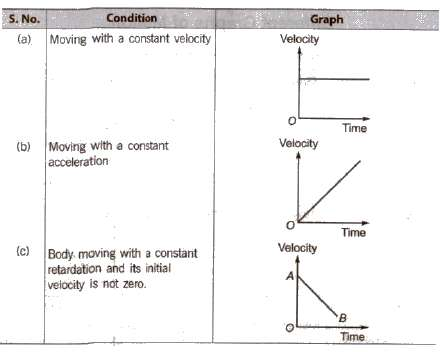#MOTION IN A STRAIGHT LINE

## Motion

If an object changes its position with respect to its surroundings with time, then it is called in motion.

Rest

If an object does not change its position with respect to its surroundings with time, then it is called at rest. [Rest and motion are relative states. It means an object which is at rest in one frame of reference can be in motion in another frame of reference at the same time.]

Point Mass Object An object can be considered as a point mass object, if the distance travelled by it in motion is very large in comparison to its dimensions.

## Types of Motion

### 1. One Dimensional Motion

If only one out of three coordinates specifying the position of the object changes with respect to time, then the motion is called one dimensional motion. For instance, motion of a block in a straight line motion of a train along a straight track a man walking on a level and narrow road and object falling under gravity etc.

### 2. Two Dimensional Motion

If only two out of three coordinates specifying the position of the object changes with respect to time, then the motion is called two dimensional motion. A circular motion is an instance of two dimensional motion.

### 3. Three Dimensional Motion

If all the three coordinates specifying the position of the object changes with respect to time, then the motion is called three dimensional motion. A few instances of three dimension are flying bird, a flying kite, a flying aero plane, the random motion of gas molecule etc.

## Distance

The length of the actual path traversed by an object is called the distance. It is a scalar quantity and it can never be zero or negative during the motion of an object. Its unit is metre.

Displacement

The shortest distance between the initial and final positions of any object during motion is called displacement. The displacement of an object in a given time can be positive, zero or negative. It is a vector quantity. Its unit is metre.

## Speed

The time rate of change of position of the object in any direction is called speed of the object. Speed (v) = Distance travelled (s) / Time taken (t)

Its unit is m/s. It is a scalar quantity. Its dimensional formula is [M0T-1].

### Uniform Speed

If an object covers equal distances in equal intervals of time, then its speed is called uniform speed.

Non-uniform or Variable Speed

If an object covers unequal distances in equal intervals of time, then its speed is called nonuniform or variable speed.

### Average Speed

The ratio of the total distance travelled by the object to the total time taken is called average speed of the object.

Average speed = Total distanced travelled / Total time taken

If a particle travels distances s1, s2, s3 , … with speeds v1, v2, v3, …, then

Average speed = s1 + s2 + s3 + ….. / (s1 / v1 + s2 / v2 + s3 / v3 + …..)

If particle travels equal distances (s1 = s2 = s) with velocities v1 and v2,

then Average speed = 2 v1 v2 / (v1 + v2)

If a particle travels with speeds v1, v2, v3, …, during time intervals t1, t2, t3,…,

then Average speed = v1t1 + v2t2 + v3t3 +… / t1 + t2 + t3 +….

If particle travels with speeds v1, and v2 for equal time intervals, i.e., t1 = t2 = t3,

then Average speed = v1 + v2 / 2 When a body travels equal distance with speeds V1 and V2, the average speed (v) is the harmonic mean of two speeds. 2 / v = 1 / v1 + 1 / v2

### Instantaneous Speed

When an object is travelling with variable speed, then its speed at a given instant of time is called its instantaneous speed.

## Velocity

Tlie rate of change of displacement of an object in a particular direction is called its velocity.

Velocity = Displacement / Time taken Its unit is m/s.

Its dimensional formula is [M0T-1].

It is a vector quantity, as it has both, the magnitude and direction. The velocity of an object can be positive, zero and negative.

### Uniform Velocity

If an object undergoes equal displacements in equal intervals of time, then it is said to be moving with a uniform velocity.

Non-uniform or Variable Velocity

If an object undergoes unequal displacements in equal intervals of time, then it is said to be moving with a non-uniform or variable velocity.

### Relative Velocity Relative

velocity of one object with respect to another object is the time rate of change of relative position of one object with respect to another object.

Relative velocity of object A with respect to object B VAB = VA – VB

When two objects are moving in the same direction, thenWhen two objects are moving in opposite direction, thenWhen two objects are moving at an angle,

then and tan β = vB sin θ / vA – vB cos θ

### Average Velocity

The ratio of the total displacement to the total time taken is called average velocity. Average velocity = Total displacement / Total time taken Acceleration

## Acceleration

The time rate of change of velocity is called acceleration.

Acceleration (a) = Change in velocity (Δv) / Time interval (Δt)
Its unit is
m/s2

Its dimensional formula is [M0LT-2].

It is a vector quantity.
Acceleration can be positive, zero or negative. Positive acceleration means velocity increasing
with time, zero acceleration means velocity is uniform while negative acceleration (retardation)
means velocity is decreasing with time.

If a particle is accelerated for a time t1 with acceleration a1 and for a time t2 with acceleration
a2, then average acceleration

Aav = a1t1 + a2t2 / t1 + t2

Different Graphs of Motion

## Velocity – Time GraphNote Slope of velocity-time graph gives average acceleration.

## Equations of Uniformly Accelerated Motion

If a body starts with velocity (u) and after time t its velocity changes to v, if the uniform acceleration is a and the distance travelled in time t in s, then the following relations are obtained, which are called equations of uniformly accelerated motion.

(i) v = u + at

(ii) s = ut + at2

(iii) v2 = u2 + 2as

(iv) Distance travelled in nth second.

Sn = u + a / 2(2n – 1)

If a body moves with uniform acceleration and velocity changes from u to v in a time interval,
then the velocity at the mid point of its path

√u2 + v2 / 2

## Motion Under Gravity

If an object is falling freely (u = 0) under gravity, then equations of motion

(i) v = u + gt

(ii) h = ut + gt2

(iii) V2 = u2 + 2gh

Note If an object is thrown upward then g is replaced by – g in above three equations.
It thus follows that

(i) Time taken to reach maximum height
tA = u / g = √2h / g

(ii) Maximum height reached by the body

hmax = u2 / 2g

(iii) A ball is dropped from a building of height h and it reaches after t seconds on earth. From
the same building if two ball are thrown (one upwards and other downwards) with the same
velocity u and they reach the earth surface after t, and t2 seconds respectively, then

t = √t1t2

(iv) When a body is dropped freely from the top of the tower and another body is projected
horizontally from the same point, both will reach the ground at the same tim
e.

## DOWNLOAD FULL PDF Physics Notes Class 11 CHAPTER 3 MOTION IN A STRAIGHT LINE

THANK YOU VISIT AND SHARE...